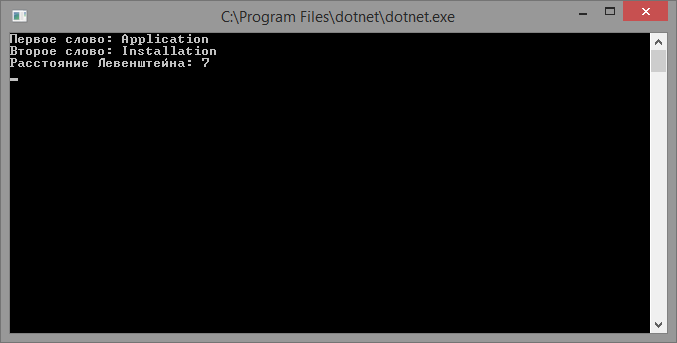# Levenshtein distance

Updated: 15.09.2019

Levenshtein distance (editorial distance, editing distance) - the minimum number of operations of deleting, inserting and replacing a character necessary to convert one line to another.

## Description of the algorithm

To find the Levenshtein distance, most often they use an algorithm in which it is necessary to fill in the D matrix with the size n + 1 by m + 1, where n and m are the lengths of the compared lines A and B according to the following rules:

• D 0,0 = 0
• D i, j = Minimum of:
• D i-1, j-1 + 0 (characters are the same)
• D i, j-1 + w delete (delete character)
• D i-1, j + w insert (insert character)
• D i-1, j-1 + w replace (character replacement)

w delete , w insert , w replace - price or weight of deleting, inserting and replacing a character.

Moreover, D n-1, m-1 - contains the value of the Levenshtein distance.

Filling the matrix for the words SATURDAY and MONDAY we get:

 S A T U R D A Y 0 1 2 3 4 5 6 7 8 M 1 1 2 3 4 5 6 7 8 O 2 2 2 3 4 5 6 7 8 N 3 3 3 3 4 5 6 7 8 D 4 4 4 4 4 5 5 6 7 A 5 5 4 5 5 5 6 5 6 Y 6 6 5 5 6 6 6 6 5

## Implementation of the algorithm

First you need to write a method to get the minimum of three numbers. The easiest way to do this is using the ternary operator:

``````static int Minimum (int a, int b, int c) => (a = a < b ? a : b) < c ? a: c;
``````
Record in classic form

### Iterative implementation

``````static int LevenshteinDistance (string firstWord, string secondWord)
{
var n = firstWord.Length + 1;
var m = secondWord.Length + 1;
var matrixD = new int [n, m];

const int deletionCost = 1;
const int insertionCost = 1;

for (var i = 0; i < n; i ++)
{
matrixD [i, 0] = i;
}

for (var j = 0; j < m; j ++)
{
matrixD [0, j] = j;
}

for (var i = 1; i < n; i ++)
{
for (var j = 1; j < m; j ++)
{
var substitutionCost = firstWord [i - 1] == secondWord [j - 1]? 0-1

matrixD [i, j] = Minimum (matrixD [i - 1, j] + deletionCost, // delete
matrixD [i, j - 1] + insertionCost, // insert
matrixD [i - 1, j - 1] + substitutionCost); // replacement
}
}

return matrixD [n - 1, m - 1];
}
``````

### Recursive implementation

``````static int LevenshteinDistance (string text1, int len1, string text2, int len2)
{
if (len1 == 0)
{
return len2;
}

if (len2 == 0)
{
return len1;
}

var substitutionCost = 0;
if (text1 [len1 - 1]! = text2 [len2 - 1])
{
substitutionCost = 1;
}

var deletion = LevenshteinDistance (text1, len1 - 1, text2, len2) + 1;
var insertion = LevenshteinDistance (text1, len1, text2, len2 - 1) + 1;
var substitution = LevenshteinDistance (text1, len1 - 1, text2, len2 - 1) + substitutionCost;

return Minimum (deletion, insertion, substitution);
}

static int LevenshteinDistance (string word1, string word2) =>
LevenshteinDistance (word1, word1.Length, word2, word2.Length);
``````

## Program for finding Levenshtein distance

In the program class you need to put a method for finding the minimum value and one of the implementations of the algorithm, and in the body of the Main method the following code^

``````static void Main (string [] args)
{
Console.Write ("First word:");
The result of the program: